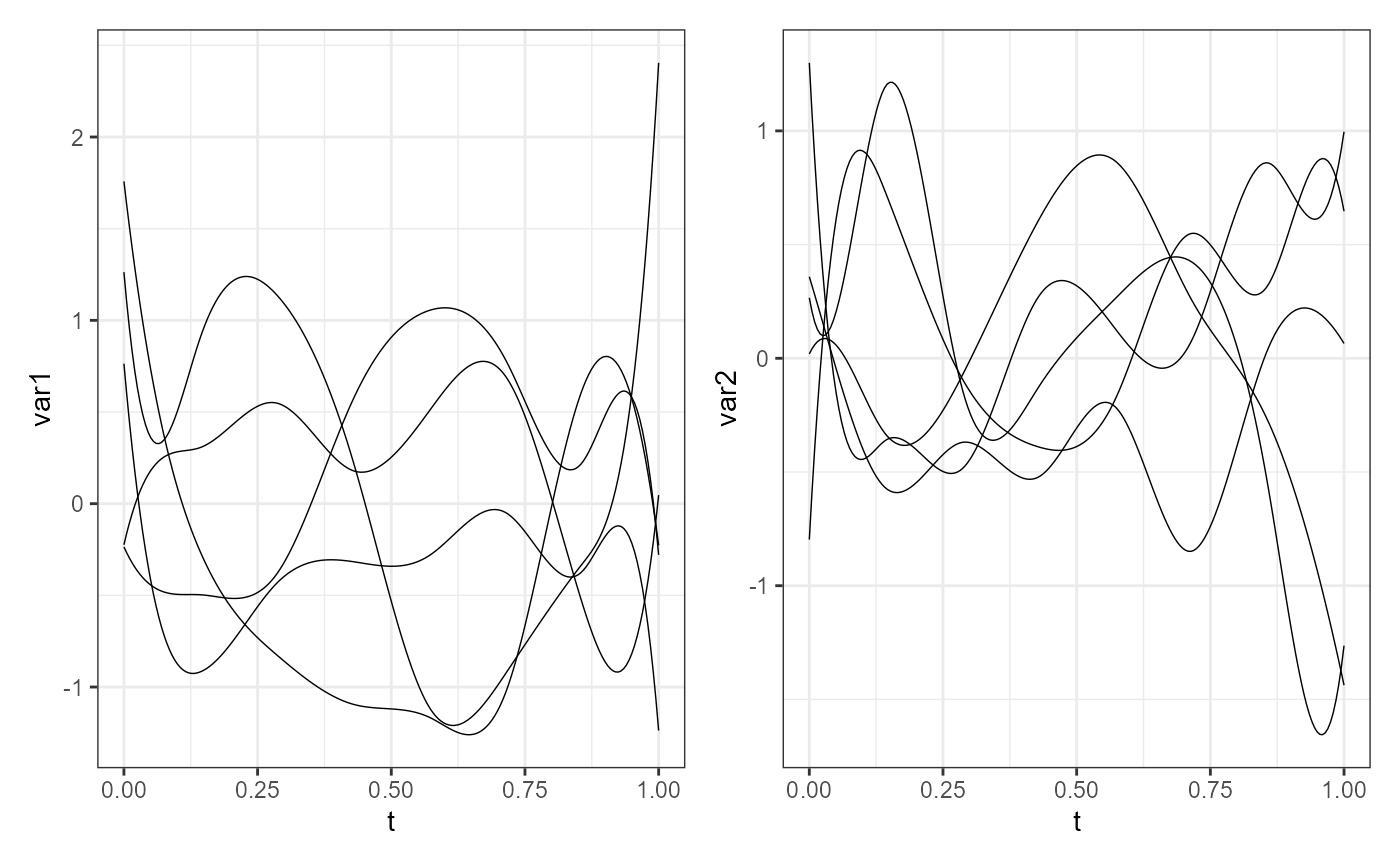This is the constructor function for objects of the mfd class. It is a wrapper to fda::fd, but it forces the coef argument to be a three-dimensional array of coefficients even if the functional data is univariate. Moreover, it allows to include the original raw data from which you get the smooth functional data. Finally, it also includes the matrix of precomputed inner products of the basis functions, which can be useful to speed up computations when calculating inner products between functional observations

mfd(coef, basisobj, fdnames = NULL, raw = NULL, id_var = NULL, B = NULL)

## Arguments

coef

A three-dimensional array of coefficients:

* the first dimension corresponds to basis functions.

* the second dimension corresponds to the number of multivariate functional observations.

* the third dimension corresponds to variables.

basisobj

A functional basis object defining the basis, as provided to fda::fd, but there is no default.

fdnames

A list of length 3, each member being a string vector containing labels for the levels of the corresponding dimension of the discrete data.

The first dimension is for a single character indicating the argument values, i.e. the variable on the functional domain.

The second is for replications, i.e. it denotes the functional observations.

The third is for functional variables' names.

raw

A data frame containing the original discrete data. Default is NULL, however, if provided, it must contain:

a column (indicated by the id_var argument) denoting the functional observations, which must correspond to values in fdnames[],

a column named as fdnames[], returning the argument values of each function

as many columns as the functional variables, named as in fdnames[], containing the discrete functional values for each variable.

id_var

A single character value indicating the column in the raw argument containing the functional observations (as in fdnames[]), default is NULL.

B

A matrix with the inner products of the basis functions. If NULL, it is calculated from the basis object provided. Default is NULL.

## Value

A multivariate functional data object (i.e., having class mfd), which is a list with components named coefs, basis, and fdnames, as for class fd, with possibly in addition the components raw and id_var.

## Details

To check that an object is of this class, use function is.mfd.

## Examples

library(funcharts)
library(fda)
set.seed(0)
nobs <- 5
nbasis <- 10
nvar <- 2
coef <- array(rnorm(nobs * nbasis * nvar), dim = c(nbasis, nobs, nvar))
bs <- create.bspline.basis(rangeval = c(0, 1), nbasis = nbasis)
mfdobj <- mfd(coef = coef, basisobj = bs)
plot_mfd(mfdobj)